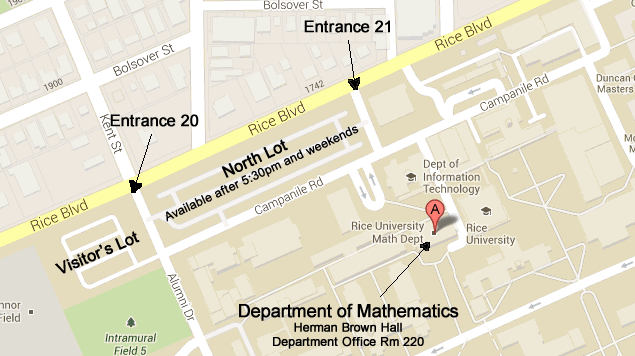## Dynamical systems and ergodic theory

### Faculty

• David Damanik

Spectral theory, mathematical physics, and analysis.

• Milivoje Lukic

Schröedinger operators and nonlinear integrable partial differential equations.

### Instructors

• Gökalp Alpan

Orthogonal polynomials, potential theory, fractal geometry, complex dynamics.

• Selim Sukhtaiev

Mathematical physics, partial differential equations, spectral theory, infinite dimensional dynamical systems.Large Map

Tel (713) 348-4829